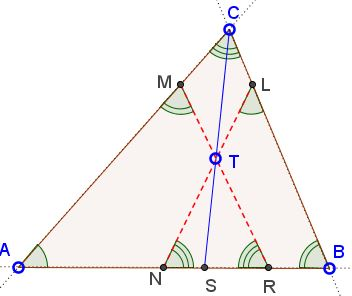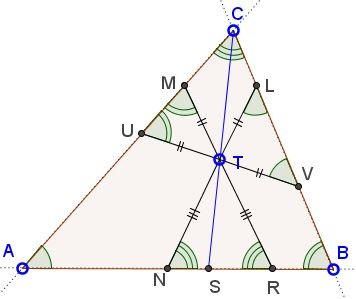# Symmedian and 2 AntiparallelsWhat is this about? A Mathematical Droodle

ExplanationThe applets illustrates the following statement:

In a triangle ABC the antiparallels to sides AB and AC that meet on the symmedian from C have equal lengths.Let CS be the symmedian and MR and LN the two antiparallels in question that meet in point T on CS. Triangle RTN having equal base angles at R and N is isosceles. Therefore,

 (1) TN = TR.

Draw the third antiparallel UV through T.Similarly to the above, we have

TL = TV and
TM = TU.

However, as we know,

TU = TV.

Therefore

 MR = TM + TR = TL + TN = LN.

Note that we actually got a little more than claimed: the corresponding pieces of the equal antiparallels cut off by the symmedian are also equal.

By transitivity, the three antiparallels through the symmedian point all have equal lengths.### Symmedian•